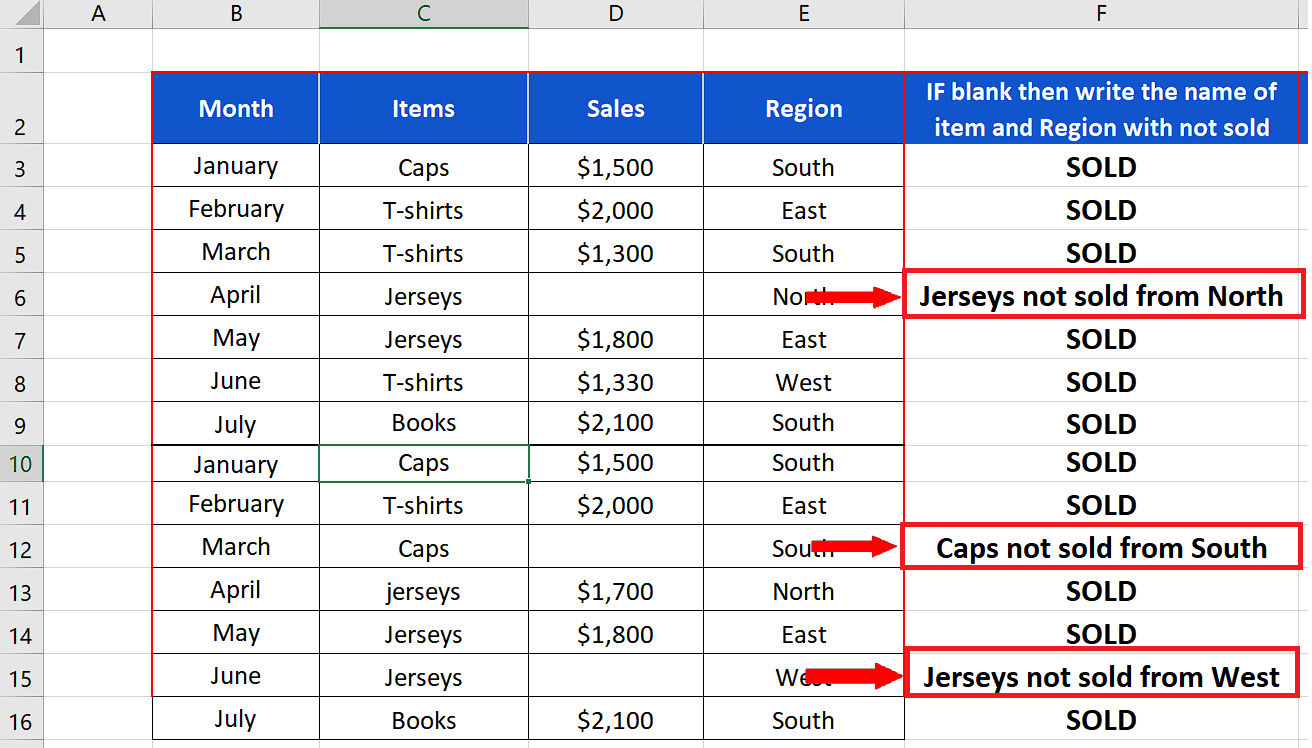# How to determine if a cell is blank in Excel

Sometimes when you are working with big datasets, you need to determine if the cells are blank or not. Excel provides us with a built-in function to check whether the cell is blank or otherwise through ISBLANK function. We can also use ISBLANK with conditional formatting to highlight the blank cells. We can also count the number of blank cells to get an idea how many cells are blank in our dataset by using another function COUNTBLANK.

In the data set shown above we can see that we have 58 rows of data and there are blanks in the data as well. Imagine if this sort of data expands to 1000s of rows, then visually finding the blanks can be almost impossible. So, let’s use Excel effectively to determine the blanks and then count them by following the steps mentioned below.

## Syntax of ISBLANK

The syntax of ISBLANK function is as follows;

ISBLANK(value)

value:

It represents the cell which is to be checked for being blank. If the cell is blank a TRUE will be returned and a FALSE will be returned in the other case. So, we will use ISBLANK with IF function to determine and write a meaningful message about the blank cells in our tutorial.

## Syntax of COUNTBLANK

The syntax of ISBLANK function is as follows;

COUNTBLANK(range)

range: It represents the range of cells which is to be checked for being blank. Excel will automatically count the blanks and return a number representing the blanks in the data range.Excel has a wide variety of functions and tools that help us to visualize the data for better analysis. Especially if we are working with large datasets then better visualization and representation makes it easier to analyze the data.

### Step 1 – Create the appropriate formula with IF and ISBLANK– We’ll use the following formula in an appropriate cell to determine if a cell is blank and then display a meaningful message as shown in the image below.
=IF(ISBLANK(D3), C3&” not sold from “&E3,”SOLD”)

### Step 2 – Count the cells if blank– We’ll use the following formula in an appropriate cell to count the number of cells which are blank as shown in the image below
=COUNTBLANK(B3:E16)

## Breakdown of the formula used in Step 1

=IF(ISBLANK(D3), C3&” not sold from “&E3,”SOLD”)

The formula makes use of ISBLANK to determine if the cell is blank then it uses the IF condition to create a useful message so that we know why a cell is empty in the sales column. Cell C3 in the formula represents the name of the item which is combined with the text “ not sold from “ using & ampersand sign and combined with the region name available in cell E3. So, whenever a blank cell is found e.g., in D6 then we’ll get this message Jerseys not sold from North. If the cell is not blank then we’ll get the message SOLD.